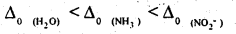# 2nd PUC Chemistry Question Bank Chapter 9 Coordination Compounds

## Karnataka 2nd PUC Chemistry Question Bank Chapter 9 Coordination Compounds

### 2nd PUC Chemistry Coordination Compounds NCERT Textbook Questions and Answers

Question 1.
Explain the bonding in coordination compounds in terms of Werner’s postulates.
Werner’s postulates explain the bonding in coordination compounds as follows:
(i) A metal exhibits two types of valencies ‘ namely, primary and secondary valencies.
Primary valencies are satisfied by negative ions while secondary valences are satisfied by both negative and neutral ions.
(In modem terminology the primary valency corresponds to the oxidation number of the metal ion, whereas the secondary valency refers to the coordination number of the metal ion)

(ii) A metal ion has a definite number of secondary valences around the central atom. Also, these valences project in a specific direction in the space assigned to the definite geometry of the coordination compound.

(iii) Primary valences are usually ionisable, while secondary valences are non ionisable.

Question 2.
FeSO4 solution mixed with (NH4)2SO4 solution in 1:1 molar ratio gives the test of Fe2+ ion but CuSO4 solution mixed with aqueous ammonia in 1:4 molar ratio does not give the test of Cu2+ ion. Explain why?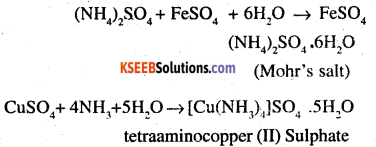Both compounds i.e. FeSO4 (NH4)2SO4.6H2O and [Cu(NH3)4]SO4.5H2O fall under the category of addition compounds with only one major difference i.e. the former is an example of a double salt, while the latter is a coordination compound. A double salt is an addition compound that is stable in the solid state bnK that which breaks up into its constituent ions in the dissolved state. These compounds exhibit individual properties of their constituents for e.g. FeSO4 (NH4)2SO4.6H2O breaks into Fe2+ NH4+, and SO42- ions. Hence, it gives a positive test for Fe2+ ions. A coordination compound is an addition compound which retains its identity In the solid as well as in the dissolved state. However, the individual properties of the constituents are cost. This happens because [Cu(NH3)]4]SO4.5H2O does not show the test for Cu2+ The ions present in the solution of [Cu(NH3)4]SO4.5H2O are [Cu(NH3)4]2+ and SO42-Question 3
Explain with two examples each of the following: coordination entity, ligand, coordination number, coordination polyhedron, homoleptic and heteroleptic complexes;
(i) Coordination entity:
A coordination entity is an electrically charged radical or species carrying a positive or negative charge. In a coordination entity, the central atom or ion is surrounded by a suitable number of neutral molecules or negative ion (called, ligands).
For examples:
[Ni(NH3)6]2+, [Fe(CN)6]4+ = cationic complex
[PtCl4]2-, [Ag(CN)2] – anionic complex
[Ni (CO)4], [CO (NH3)4Cl2] = neutral complex

(ii) ligand:
The neutral molecules or negatively charged ions that surround the metal atom in a coordination entity or a coordinal complex are known as ligands.
For example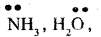Cl, OH. Ligands are usually polar in nature and possess at least one unshared pair of valence electrons.

(iii) Coordination number:
The total number of ligands (either neutral molecules or negative ions) that get attached to the central metal atom in the coordination sphere is called the coordination number of the central metal atom. It is also referred to as its ligaricy.
For example:
(a) In the complex K2[PtCl6], there are six chloride ions attached to Pt in the coordinate sphere. Therefore, the coordination number of Pt is 6.
(b) similarly, in the complex [Ni (NH3)4 Cl2], the cooordination number of the central atom (Ni) is 6.

(iv) Coordination polyhedron:
Coordination polyhedrons, about the central atom can be defined as the spatial arrangement of the ligands that are directly attached to the central metal ion in the coordination sphere.
For example:
(a) Square planar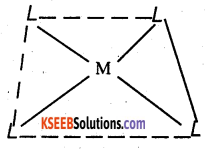(b) Square planar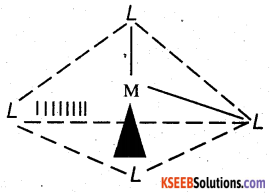(v) Homoleptic complexes:
These are those complexes in which the metal ion is bound to only one kind of a donor group For e.g.:
[CO (NH3)6]3+
[pt Cl4]2

(vi) Heteroleptic complexes:
Heteroleptic complexes are those complexes where the central metal is bound to more than one type of a donor group.
For e.g.:
[CO(NH3)4C2]+
[CO(NH3)5 Cl]2+

Question 4.
What is meant by unidentate, didentate and ambidentate ligands? Give two examples for each.
A ligand may contain one or more unshared pairs of electrons which are called the donor sites of ligands. Now depending on the number of these donor sites, ligands can be classified as follows.
(a) Unidentate ligands: Ligands with only one donor sites are called unidentate ligands.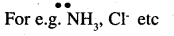(b) Didentate ligands: Ligands that have two donor sites are called didentate ligands.
For e.g.:
(a) Ethane – 1, 2 – diamine.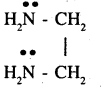(b) enolic acid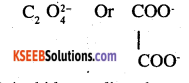c) Ambidentate ligand:
Ligands that can attach themselves to the central metal atom through two different atoms are called ambidentate ligands.
For Example :
(a)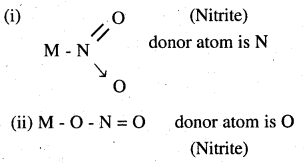(b)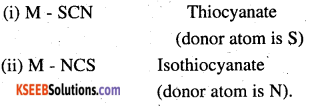Question 5.
Specify the oxidation numbers of the metals in the following coordination entities:
(i) [CO(H2O)(CN)(en)2]2+
(ii) [COBr2(en)2]+
(iii) [PtCl4]2-
(iv) K3[Fe(CN)6]
(v) [Cr(NH3)3Cl3]
(i) [CO(H2O) (CN) (en)2]2+
Let the oxidation number of CO be x.
The charge on the complex is + 2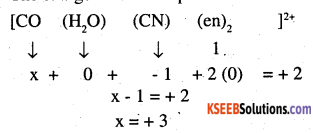(ii) [PtCl4]2-
x + 4 (-1) = -2 x = +2

(iii) [COBr2 (en)2]+
x + 2 (-1) + 2.(0) = + 1
x = + 3

(iv) K3[Fe(CN)6]
x + 6 (-1) = -3
x = + 3

(v) [Cr (NH3)3 Cl3]
x + 3 (0) +3 (-1) = 0
x – 3 = 0
x = 3Question 6.
Using IUPAC norms write the formula for the following:
(i) Tetrahydroxozincate (II)
(iii) piamminedichloridoplatinum(II)
(iv) Potassium tetracyanonickelate(II)
(v) Pentaamminenitrito-O-cobalt(III)
(vi) Hexaamminecobalt(III) sulphate
(vii) Potassium tri(oxalato)chromate(III) (viii) Hexaammineplatinum(IV)
(ix) Tetrabromidocuprate(II)
(x) Pentaamminenitrito-N-cobalt(III)
(i) [Zn (O4)]2-
(ii) K2 [Pd Cl4]
(iii) [Pt (NH3)2 Cl2]
(iv) K2[Ni(CN)4]
(v) [CO (NH3)5 (ONO)]2+
(vi) [CO (NH3)6]2(SO4)3
(vii) K3 [Cr (C2O4)3]
(viii) [Pt (NH3)6]4+
(ix) [Cu (Br4)2-
(x) [CO (NO2) (NH3)5]2+

Question 7.
Using IUPAC norms write the systematic names of the following:
(i) [CO(NH3)6]Cl3
(ii) [Pt(NH3)2Cl(NH2CH3)]Cl
(iii) [Ti(H2O)6]3+
(iv) [CO(NH3)4Cl(NO2)]Cl
(v) [Mn(H2O)6]2+
(vi) [NiCl4]2-
(vii) [Ni(NH3)6]Cl2
(viii) [CO(en)3]3+
(ix) [Ni(CO)4]
(i) Hexaamminecobalt (III) chloride.
(ii) Diamine chlorido (methylamine) platinum (II) chloride.
(iii) Hexaaquatitanium (III) ion.
(iv) Tetraamminichloridonitrite – N – cobalt (HI) chloride.
(v) Flexaquamanganese (II) ion.
(vi) Tetrachloridononickeiate (II) ion.
(vii) Hexaammmenickei (11) chloride,.
(viii)Tris (ethane -1,2 – diammine) cobalt (III) ion.
(ix) Tetracarbonylnickel (O)

Question 8.
List various types of isomerism possible for coordination compounds, giving an example of each.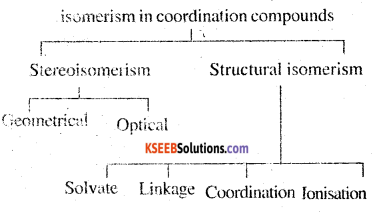(a) Geometric isomerism:
This type of isomerism is common in heteroleptic complexes It arises dire to the different possible geometric arrangements of the li sands.
For Example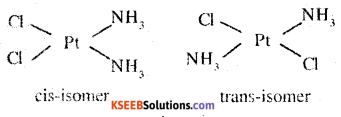(b) Optical isomerism:
This type of isomerism arises in chiral molecules Isomers are mirror images of each other and are non-superimposable.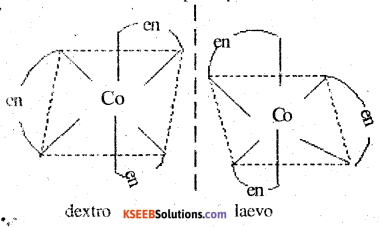This type of isomerism is found in complexes that contain ambidentate ligands.
For example:
[CO (NH3)5 (NO2)]Cl2 and
[CO (NH3)5 (ONO)] Cl2

(d) Coordination isomerism:
This type of isomerism arises when the ligands are inter changed between cationic and anionic entities of different metal ions present in the complex.
[CO (NH3)6] [Cr (CN)6] and
[CO (CN)6][Cr (CN)6]

(e) Ionisation isomerism:
This type of isomerism arises when a counter ion replaces a ligand within the coordination sphere. Thus, complexes that have the same composition, but furnish different ions when dissolved in water are called ionisation isomers.
For example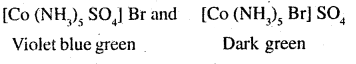Question 9.
How many geometrical isomers are possible in the following coordination entities?
(i) [Cr(C2O4)3]3-
(ii) [CO(NH3)3Cl3]
(i) For [Cr(C2O4)3]3- No geometric isomer is possible as it is a bidentate ligand.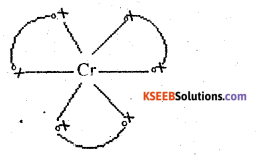(ii) [CO(NH3)3Cl3]
Two geometrical isomers are possible.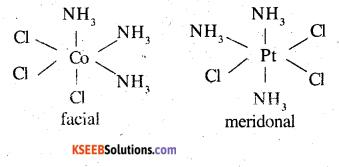Question 10.
Draw the structures of optical isomers of:
(1) [Cr(C2O4)3]3-
(ii) [PtCl2(en)2]2+
(iii) [Cr(NH3)2Cl2(en)]+
(i) [Cr(C2O4)3]3-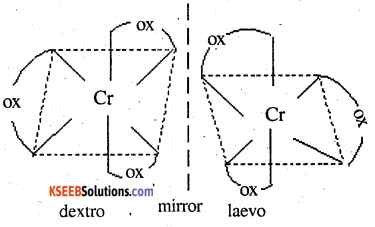(ii) [PtCl2(en)2]2+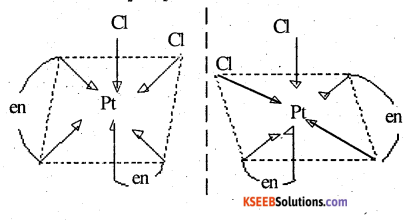(iii) [Cr(NH3(en)2]2+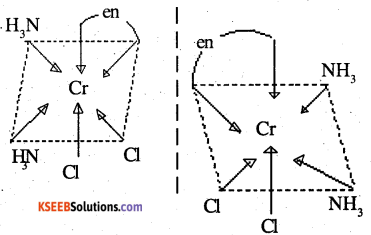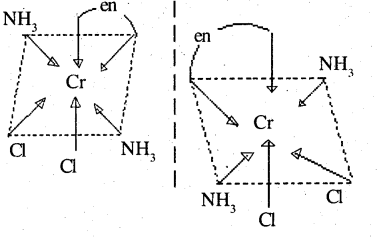Question 11.
Draw all the isomers (geometrical and optical) of:
(i) [COCl2(en)2]+
(ii)[CO(NH3)Cl(en)2]2+
(iii) [CO(NH3)2Cl2(en)]+
(i) [COCl2(en)2]+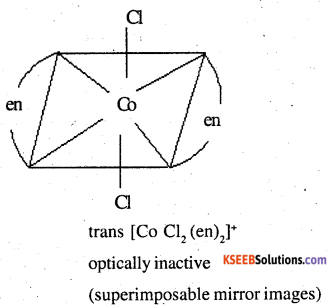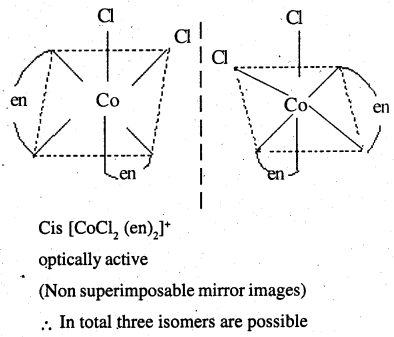(ii)[CO(NH3)Cl(en)2]2+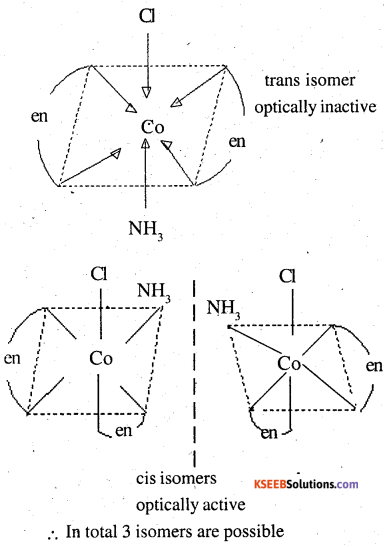(iii) [CO(NH3)2Cl2(en)]+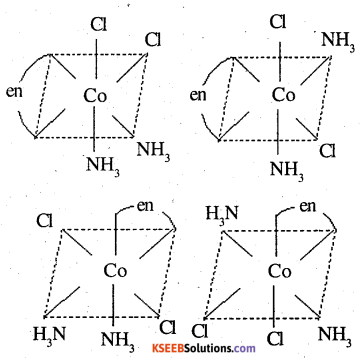Question 12.
Write all the geometrical isomers of [Pt(NH3)(Br)(Cl)(py)] and how many of these will exhibit optical isomers?
[Pt (NH3) (Br) (eC) (Pg)]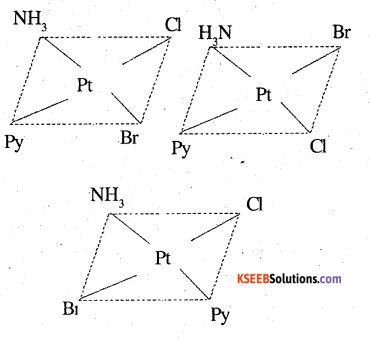From the above isomers, none will exhibit optical isomers. Tetrahedral complexes rarely show optical isomerisation. They do so only in presence of unsymmetric chelating agents.

Question 13.
Aqueous copper sulphate solution (blue in colour) gives:
(i) a green precipitate with aqueous potassium fluoride and
(ii) a bright green solution with aqueous potassium chloride. Explain these experimental results.
Aqueous CuSO4 exists as [Cu(H2O)4]SO4. It is blue in colour due to presence of [CU(H2O)4]2+ ions.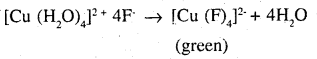[Cu (H2O)4]2+ 4Cl → [CuCl4]2- + 4H2O
In both the cases, the weak field ligand water is replaced by the F and Cl ions.Question 14.
What is the coordination entity formed when excess of aqueous KCN is added to an aqueous solution of copper sulphate? Why is it that no precipitate of copper sulphide is obtained when H2S(g) is passed through this solution?
CuSO4(aq) + 4KCN (aq) → K2Cu (CN)4](aq) + K2SO4
i.e. [Cu (H2O)4]2+ + 4CN → [Cu (CN)4]2- + 4H2O
Thus, the coordination entity formed in the process is K2 [Cu (CN)4]. K2 [Cu (CN)4] is a very stable complex which does not ionize to give Cu2+ ions when added to water. Hence Cu2+ ions are not precipitated when H2S(g) is passed through the solution.

Question 15.
Discuss the nature of bonding in the following coordination entities on the basis of valence bond theory:
(i) [Fe(CN)6]4-
(ii) [FeF6]3-
(Hi) [CO(C2O4)3]3-
(iv) [COF6]3-
(i) [Fe(CN)6]4-
In the above coordination complex. Iron exists in +2 oxidation state.
Fe2+: electronic configuration is 3d6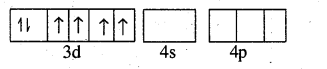As CN is a strong field ligand, it causes pairing of the unpaired 3d electrons.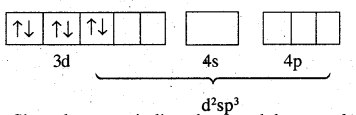Since there are six ligands aound the central metal ion, the most feasible hybridisation is d2sp3 6 electrons pairs from CN ions occupy the six hybrid d2sp3 orbitais .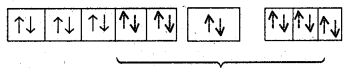Hence, the geometry of the complex is octahedral and the complex is diamagnetic (as there are no unpaired electrons).

(ii) [FeF6]3-
Oxidation state of Fe is + 3
Orbitais of Fe+3 ion.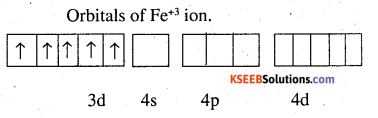There are 6F ions. Thus, it will undergo d2Sp3 or sp3d2 hybridisation. As F is a weak ligand, it does not cause pairing of electrons in the 3d orbitals. Hence the most feasible hybridisation is Sp3d2.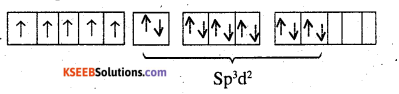Hence the geometry of the complex is found to be octahedral.

(iii) [CO(C2O4)3]3-
Cobalt exists + 3 oxidation state in the complex.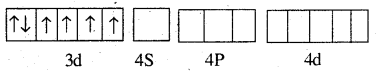Oxalate is a weak field ligand. Therefore, it cannot cause pairing of the 3d orbital electrons.
As there are 6 ligands, hybridisation has to be either Sp3d2 or d2Sp3 hybridization.
As there are no pairing of electrons Sp3d2 is the most stable hybridisation.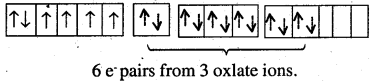Oxalate being a bidentate ligand donates 2 pairs of electrons each.
Hence, the geometry of the complex is found to be octahedral.

(iv) [COF6]3-
cobalt exists in the +3 oxidation state.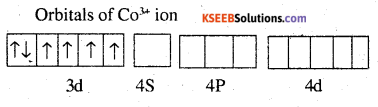Again, fluoride ion is a weak ligand. It cannot cause the pairing of the 3d electrons. Sp3d2 hybridised orbitals of CO3+ ion are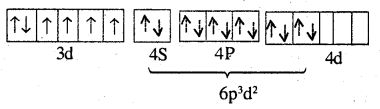Hence, the geometry of the complex is octahedral and paramagnetic.Question 16.
Draw figure to show the splitting of d orbitals in an octahedral crystal field.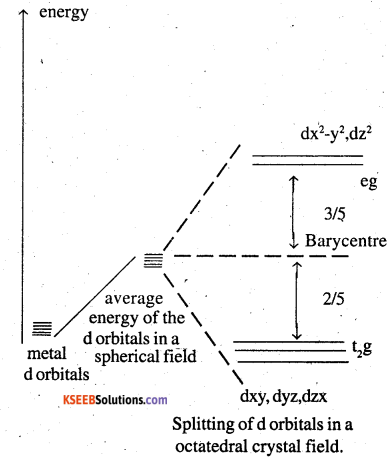The splitting of d orbitals in an octahedral field takes place in such a way that dx2-y2, dz2 experience a rise in energy and form the e.g. level, dry, dyz, dzx experience a fall in energy and form t2g level.

Question 17.
What is spectrochemical series? Explain the difference between a weak field ligand and a strong field ligand.
A spectrochemical series is the arrangement of common ligands in the increasing order of their crystal field splitting energy (CSFE) values the ligands present on the RHS of the series are strong fields ligands while that on the LHS are weak field ligands. Also, strong field ligands cause higher splitting in the d orbitals than weak field ligands.
I < Br < S2- < SCN< Cl < N3 < F < OH< C2O42- ~ H2O
< NCS ~ H < CN < NH3, < en ~ SO32-2- < NO2 < phen < COQuestion 18.
What is crystal field splitting energy? How does the magnitude of ∆o decide the actual configuration of d orbitals in a coordination entity?
The degenerate d-orbitals (in a spherical field environment) split two level i.e. eg and t2g in the presence of ligands. The splitting of the degenerate orbitals in the presence of ligands is called crystal field splitting and the energy difference between the two levels (e and t2g) is called the crystal field splitting energy. It is denoted by ∆o. After the orbitals have split, the filling of the electrons takes place. After 1 electron (each) has filled in the three t2g orbitals, the filling of the electrons takes place in 2 ways.

It can enter the orbital (giving) rise to t3g eg, like electronic configuration on the pairing of the electrons can take place in the t2g orbitals
(giving rise to t42g eg0 like electronic configuration). If the ∆o value of a ligand is less than the pairing energy, then the electrons enter the eg orbital. On the other hand, if the ∆o value of a ligand is more than the pairing energy, then the electrons enter the t2g orbitals.

Question 19.
[Cr(NH3)6]3+ is paramagnetic while [Ni(CN)4]2- is diamagnetic. Explain why?
Cr is in the +3 oxidation state i.e. d3 configuration. Also, NH3 is a weak field ligand that does not cause the pairing of the electrons in the 3d orbitals.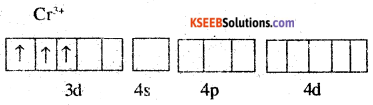Therefore, it undergoes d2sp3 hybridisation and the electrons in the 3d orbitals remain unpaired. Hence, ibis paramagnetic in nature.
In [Ni CCN)4]2-, Ni exists in the +2 oxidation state.
i.e, d8 Configuration.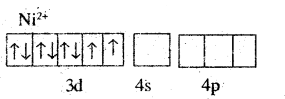CN is a strong field ligand. It causes the pairing of 3d orbital electrons. Then Ni2+ undergoes dsp2 hybridisation.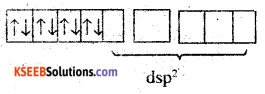As there are no unpaired ejecirons, it is diamagnetic.

Question 20.
A solution of [Ni(H2O)6]2+ is green but a solution of [Ni(CN)4]2- is colourless. Explain.
In [Ni(H2O)6]2+ is a weak field ligand. Therefore there are unpaired electrons in Ni2+. In this complex the cl electrons from the lower energy level can be excited to the higher energy level is the possib.lity of d- d transition is present. Hence [Ni(H2O)6]2+ is coloured.

In [Ni(CN)4]2- CN is a strong field ligand. Therefore, d- d transition is not possible due to absence of unpaired electron (CN initiates pairing of d elcctrons ). Hence it is colourless.

Question 21.
[Fe(CN)6]4- and [Fe(H2O)6]2- are of different colours in dilute solutions. Why?
The colour of a particular coordination compound depends on the magnitude of crystal field splitting energy ∆. This CFSE in turn depends on the nature of the ligand. In case of [Fe (CN)6)4- and [Fe (H2O)6]2- the colour differs because there is a difference in the CFSE. Now, CN is a strong field ligand having a higher CFSE value as compared to the CFSE value of water. This means that absorption of energy for the extra d- d transition also differed. Hence, the transmitted colour also different.

Question 22.
Discuss the nature of bonding in, metal carbonyls.
The metal carbon bonds in’ metal carbonyls have both σ and π characteristics. A σ bond is formed when the carbonyl carbon donates a lone pair of electrons to the vacant orbital of metal. A π bond is formed by the donation of a pair of vacant anti-bonding π orbital (also known as back bonding of the carbonyl group). The σ bond strengthens the π bond and vice-versa. This synergic effect is created due to this metal-ligand bonding. This synergic effect strengthens the bond between CO and metal.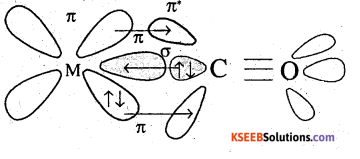Question 23.
Give the oxidation state, d orbital occupation and coordination number of the central metal ion in the following complexes:
(i) K3[CO(C2O4)3]
(ii) cis-[Cr(en)2Cl2]Cl
(iii) (NH4)2[COF4]
(iv) [Mn(H2O)6]SO4
(i) K3[CO(C2O4)3]
The central metal ion is CO
coordination number: 6
oxidation state: +3
d orbital occupation : t62g eg0

(ii) cis-[Cr(en)2Cl2]Cl
central metal: Cr
coordination number: 6
oxidation state:+3 .
d orbital occupation : t32g eg0

(iii) (NH4)2[COF4]
central metal: Co oxidation state: +2 coordination number: 4
d orbital occupation : eg4 t32g

(iv)[Mn(H2O)6]SO4
Central metal: Mn
coordination number: 6
oxidation state : + 2
d orbital occupancy : t32g eg2

Question 24.
Write down the IUPAC name for-each of the following complexes and indicate the oxidation state, electronic configuration and coordination number. Also give stereochemistry and magnetic moment of the complex:
(i) K[Cr(H2O)2(C2O4)2].3H2O
(ii) [CO(NH3)5Cl.]Cl2
(iii) CrCl3(py)3
(iv) Cs[FeCl4]
(v) K4[Mn(CN)6]
(i) Potassium diaquadioxatatochromate (III) trihydrate oxidation state of chromium = +3
Electronic configuration: 3d3: t32g
coordination number: 6
Shape: octahedral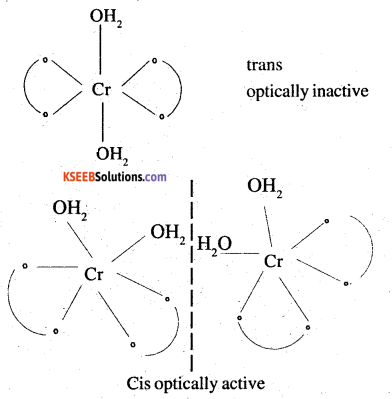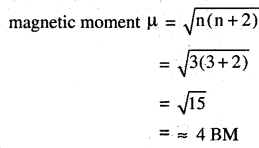(ii) [Co (NH3)5Cl]Cl2
Pentaamminechloridocobalt (III) chloride
oxidation state =+3
coordination number = 6
shape: octahedral
electronic configuration : d6: t62g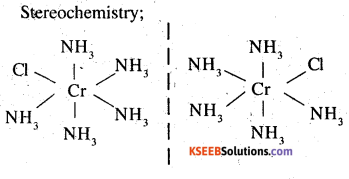Magnetic moment = 0 (∵ n = 0)

(iii) CrCl3(py)3
Trichloridopyridinechromium (III)
oxidation state = +3
electronic configuration : d3: t32g
coordihation number = 6
shape = octahedral
Stereochemistry: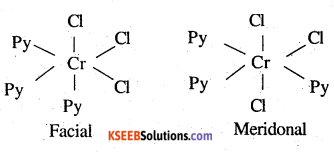Both isomers are optically active. Therefore, a total of 4 isomers exist.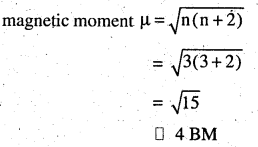(iv) Cs [Fe Cl4]
IVPAC name: Caesium tetrachloroferrate (III)
oxidation state = +3
coordination number = 4
shape: tetrahedral
stereochemistry: optically inactive
Magnetic moment(v) K4[Mn(CN)6]
Potassium hexacyanomanganate (II)
oxidation state =+2
electronic configuration = d5 : t52g
coordination number = 6
shape : octahedral
stereochemistry: optically inactive
Magnetic moment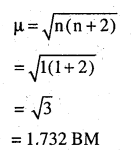Question 25.
What is meant by stability of a coordination compound in solution? State the factor^ which govern stability of complexes.
The stability of a complex in a solution refers to the degree of association between the two species involved in a state of equilibrium stability can be expressed quantitatively in terms of stability constant pr formation constant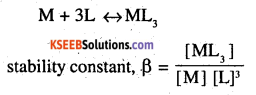For this reaction, the greater the value of the stability constant, the greater is the proportion of ML3 in the solution.
stability can be two types

(a) Thermodynamic stability:
The extent to which the complex will be formed or will be transformed into another species at the point of equilibrium is determined by thermodynamic stability.

(b) Kinetic stability:
This helps in determining the speed with which the transformation will occur to attain the state of equiftbrium.
Factors that affect the stability of a complex are: ~ ;
(i) charge on the central metal ion: The greater the charge on the central metal ion, the greater in the stability of the complex.
(ii) Basic nature of ligand: A more basic ligand will form a more stable complex.

(c) Presence of chelate rings: Chelation increases the stability of complexes

Question 26.
What is meant by the chelate effect? Give an example.
When a ligand attaches to the metal ion in a manner that form’s a ring, then the metal ligand association is found to be more stable. In other words, we can say that complexes containing chelate rings more stable than complexes without rings. This is known as chelate effect.
For example: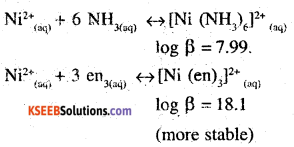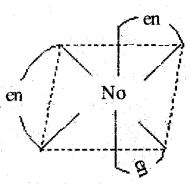Question 27.
Discuss briefly giving an example in each case the role of coordination compounds in:
(i) biological systems
(ii) medicinal chemistry and
(iii) analytical chemistry
(iv) extraction/metaliurgy of metals.
(i) Role of coordination compounds in biological systems:
We know that photosynthesis is possible by the presence of the chlorophyll pigment. This pigment is a coordination compound of magnesium. In the human biological system, several coordination compounds play important roles. For example the oxygen – carrier of blood, i.e haemoglobin is a coordination compound of iron.

(ii) Role of coordination compounds in Medicinal chemistry: Certain coordination compounds of platinum (for example cis-platin) are used for inhibiting the growth of tumors.

(iii) Role of coordination compounds in analytical chemistry: During salt analysis, a number of basic radicals are detected with the help of the colour changes they exhibit with different reagents. These colour changes are a result of the coordination compounds or complexes that the basic radicals form with different ligands.

(iv) Role of coordination compounds in interaction or metallurgy of metals: The process of extraction of some of the metals from their ores involves the formation of complexes. For example in aqueous solution, gold combines with cyanide ions to form [Au (CN)2]. From this solution, gold is later extracted by the addition of Zn metal.Question 28.
How many ions are produced from the complex CO(NH3)6Cl2 in solution?
(i) 6
(ii) 4
(iii) 3
(iv) 2
(iii) The given complex can be written as

[CO(NH3)6]Cl2 Thus, [CO (NH3)6]+ along with two Cl ions are produced.

Question 29.
Amongst the following ions which one has the highest magnetic moment value?
(i) [Cr(H2O)6]3+
(ii) [Fe(H2O)6]2+
(iii) [Zn(H2O)6]2+
( i) n = 3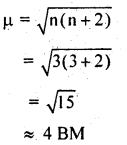(ii) n = 4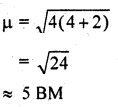(iii) n = 0
μ = 0
Hence [FeCH2O)6]2+ has the highest magnetic moment value.

Question 30.
The oxidation number of cobalt in K[CO(CO)4] is
(i) +1 (ii) +3 (iii) -1 (iv) -3
We know that CG is a neutral ligand and K carries a charge of + 1. Therefore, the complex is written as K+ [Co (CO)4]. Therefore, the oxidation number of Co in the given complex is -1.
Hence (III) is correct.

Question 31.
Amongst the following, the most stable complex is
(i) [Fe(H2O)6]3+
(ii) [Fe(NH3)6]3+
(iii) [FeiC2O4)3]3-
(iv) [FeCl6]3-
We know that the stability of a complex substance increases by chelation. Therefore, the most stable complex is [Fe (C2O4)3]3-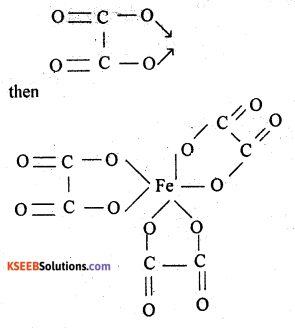Question 32.
What will be the correct order for the wavelengths of absorption in the visible region for the following:
[Ni(NO2)6]4-, [Ni(NH3)6]2+, [Ni(H2O)6]2+?